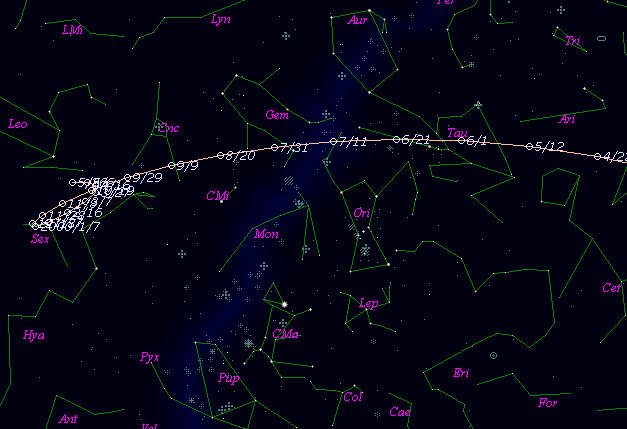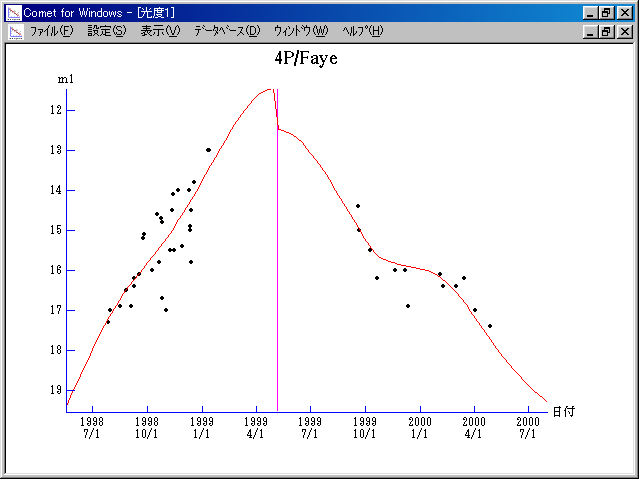# 4P/Faye (1999)###Pictures###Orbital Elements

```   The following improved orbital elements by Kenji Muraoka,
are from 349 observations 1983 to 1998, including 9 Planets,
Moon and 5 minor planets perturbations and non-gravitational
effect of style II.  The mean residual is +/- 0.83 arc seconds.

Epoch  =  1999 May  22.0  TT       JDT = 2451320.5
T  =  1999 May   6.10348       +/- 0.00035 (m.e.) TT
Peri. =  204.97434                +/- 0.00015
Node  =  199.33887                +/- 0.00006   (2000.0)
Incl. =    9.04894                +/- 0.00001
q  =    1.6570009              +/- 0.0000007 AU
e  =    0.5682548              +/- 0.0000002
a  =    3.8379141              +/- 0.0000006 AU
n  =    0.13108747             +/- 0.00000003
P  =    7.519                  +/- 0.0000018  years
A1  =   +0.546                  +/- 0.021
A2  =   -0.01936                +/- 0.00026
```

###Finding Charts###Magnitudes Graph

```        m1 =  3.8 + 5 log d + 25.0 log r  [   ,  0]  (             - 1999 May   6)
m1 =  5.3 + 5 log d + 23.0 log r  [  0,170]  (1999 May   6 - 1999 Oct. 23)
m1 = 10.0 + 5 log d + 10.0 log r  [170,   ]  (1999 Oct. 23 -             )
```##### The orbital elements are calculated by Kenji Muraoka. The charts are made with StellaNavigator Ver.2.0 for Windows (AstroArts / ASCII). The magnitudes graphs are made with Comet for Windows.